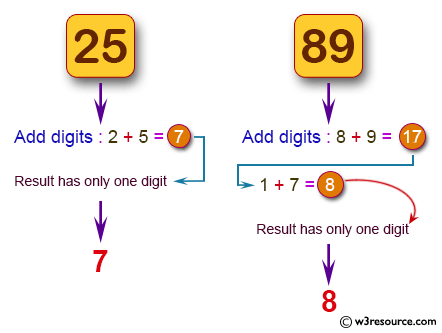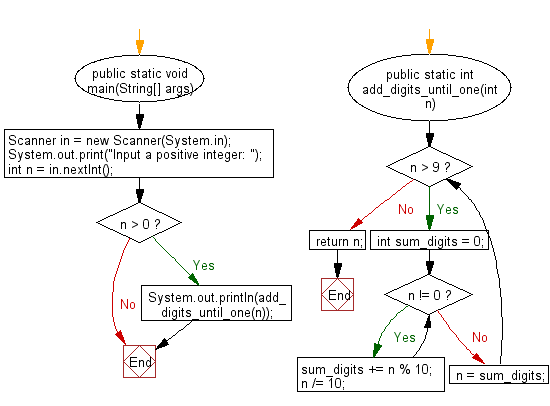﻿ Java programming exercises: Accept a positive number and repeatedly add all its digits until the result has only one digit - w3resource# Java Exercises: Accept a positive number and repeatedly add all its digits until the result has only one digit

## Java Basic: Exercise-183 with Solution

Write a Java program to accept a positive number and repeatedly add all its digits until the result has only one digit.

Pictorial Presentation:Sample Solution:

Java Code:

``````import java.util.*;
public class Solution {
public static void main(String[] args) {
Scanner in = new Scanner(System.in);
System.out.print("Input a positive integer: ");
int n = in .nextInt();
if (n > 0)
}

public static int add_digits_until_one(int n) {
while (n > 9) {
int sum_digits = 0;
while (n != 0) {
sum_digits += n % 10;
n /= 10;
}
n = sum_digits;
}
return n;
}
}
```
```

Sample Output:

```Input a positive integer:  25
7
```

Flowchart:Java Code Editor:

Company:

What is the difficulty level of this exercise?

﻿

## Java: Tips of the Day

Parsing dates:

```import java.io.*;
import java.util.*;
import java.text.*;

String s = "2001/09/23 14:39";

SimpleDateFormat formatter = new SimpleDateFormat ("yyyy/MM/dd H:mm");
Date d = formatter.parse(s, new ParsePosition(0));
```